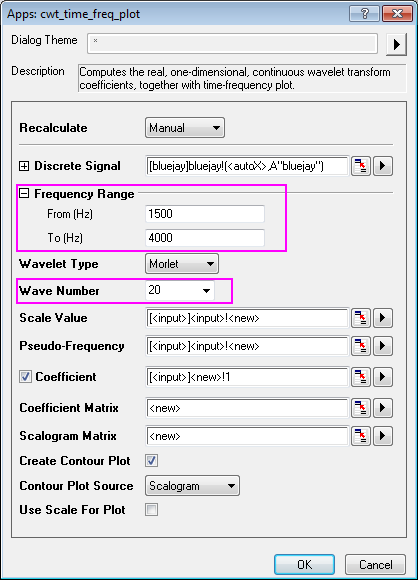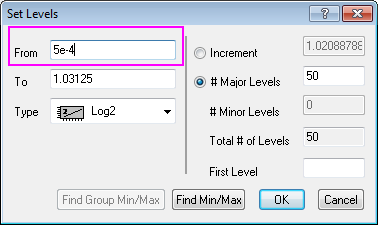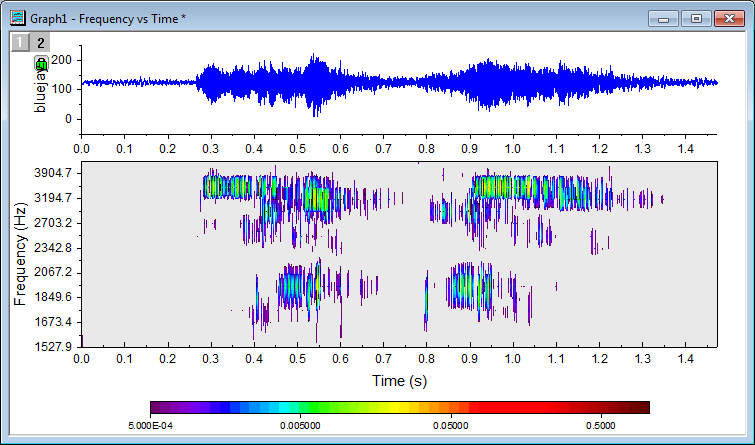#### File Exchange > Data Analysis >    Time-Frequency AnalysisAuthor:
OriginLab Technical Support
3/8/2016
Last Update:
12/21/2020
156
Total Ratings:
2
File Size:
60 KB
Average Rating:File Name:
Time-Frequ...is.opx
File Version:
1.30
Minimum Versions:
Free
Summary:

Compute one-dimensional CWT coefficients and scalogram, and create a time-frequency contour plot.

Description:

Purpose
This app can be used to perform time-frequency analysis based on continuous wavelet transform, including the following features:
1. Specify XY signal data, and get sampling interval from time (X) data automatically.
2. Specify frequency range for the continuous wavelet transform.
3. Wavelet types include Morlet, Derivative of Gaussian, and Mexican Hat.
4. Output calculated scale value and pseudo-frequency.
5. Output coefficient matrix and scalogram matrix.
6. Create graph with raw data as line plot, and coefficient or scalogram contour plot.
7. Support both scale vs time and frequency vs time contour plot.

Installation
Download the file "Time-Frequency Analysis.opx". Then drag-and-drop the file onto the Origin workspace.

Operation

• Select XY data columns from worksheet
• Click the "Time-Frequency Analysis" app icon from the Apps gallery.
• Change settings in dialog that opens, and click OK.

Example
1. With an empty worksheet activated, run the following LabTalk script in Script Window (Open by menu Window: Script Window) to import data.

fname\$ = "%@ATime-Frequency Analysis\bluejay.wav";
impWav;


2. Highlight column A of the imported data by clicking the column header, then launch the app by clicking the Time-Frequency Analysis icon from the Apps gallery.
3. In the dialog, set Frequency Range from 1500 to 4000, and Wave Number to be 20.4. Click OK button to generate the results, including scales, pseudo-frequency, virtual matrix for coefficients, coefficient matrix, scalogram matrix, and a graph with raw data plot and contour of scalogram matrix.
5. Focus on the created graph. Double click on the contour plot on the bottom layer to bring up the Plot Details dialog. Under the Colormap/Contours tab, click the Level... header of the table to set the levels, as the following image shows, setting From as 5e-4.6. Go back to Plot Details dialog and click OK button to finish the setting, and get the result graph.Algorithm

1. Continuous wavelet transform
2. Scalogram calculation
Assume coefficient matrix (calculated from continuous wavelet transform) is matCoef.
First we compute the product of matCoef multiplied by matCoef itself element by element, to generate matrix matProd.
Then we get the absolute value of each value in matProd, to generate matrix matProdAbs.
Then we calculate the sum of matProdAbs as sum.
Finally, the scalogram matrix, matScalogram is calcualted as 100*matProdAbs/sum.

 06/13/2021 lusaqu god03/31/2016 lsi This function is slow a little bit, but very easy and great. Never seen such a matched function for my request.(from the customer) Junji